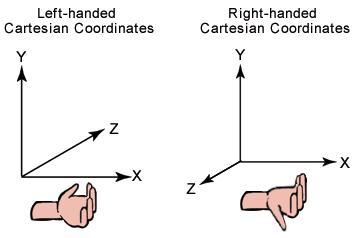# Coordinate systems

Typically 3D graphics applications use one of two types of Cartesian coordinate systems: left-handed or right-handed. In both coordinate systems, the positive x-axis points to the right, and the positive y-axis points up.

## Left and right handed coordinates

You can remember which direction the positive z-axis points by pointing the fingers of either your left or right hand in the positive x-direction and curling them into the positive y-direction. The direction your thumb points, either toward or away from you, is the direction that the positive z-axis points for that coordinate system. The following illustration shows these two coordinate systems.Direct3D uses a left-handed coordinate system. Although left-handed and right-handed coordinates are the most common systems, there is a variety of other coordinate systems used in 3D software. For example, it is not unusual for 3D modeling applications to use a coordinate system in which the y-axis points toward or away from the viewer, and the z-axis points up.

## Vertices and vectors

Given the coordinate system, an x, y and z coordinate can define a point in space (a "vertex"), or a 3D direction (a "vector").

A collection of vertices can be used to define lines and shapes. The simplest objects definable by vertices are called Primitives, and a more complex object defined by a set of primitives is called a "mesh."

The essential operations performed on meshes defined in a 3D coordinate system are translation, rotation, and scaling. You can combine these basic transformations to create a transform matrix. For details, see Transforms.

When you combine these operations, the results are not commutative; the order in which you multiply matrices is important.

## Porting from a right-handed coordinate system

If you are porting an application that is based on a right-handed coordinate system, you must make two changes to the data passed to Direct3D:

• Flip the order of triangle vertices so that the system traverses them clockwise from the front. In other words, if the vertices are v0, v1, v2, pass them to Direct3D as v0, v2, v1.
• Use the view matrix to scale world space by -1 in the z-direction. To do this, flip the sign of the _31, _32, _33, and _34 member of the matrix structure that you use for your view matrix.

Coordinate systems and geometry